Skip to content
Related Articles
Graph Plotting in Python | Set 1
• Difficulty Level : Easy
• Last Updated : 19 Feb, 2021

This series will introduce you to graphing in python with Matplotlib, which is arguably the most popular graphing and data visualization library for Python.

Installation

Easiest way to install matplotlib is to use pip. Type following command in terminal:

`pip install matplotlib`

OR, you can download it from here and install it manually.

Getting started ( Plotting a line)

 `# importing the required module``import` `matplotlib.pyplot as plt`` ` `# x axis values``x ``=` `[``1``,``2``,``3``]``# corresponding y axis values``y ``=` `[``2``,``4``,``1``]`` ` `# plotting the points ``plt.plot(x, y)`` ` `# naming the x axis``plt.xlabel(``'x - axis'``)``# naming the y axis``plt.ylabel(``'y - axis'``)`` ` `# giving a title to my graph``plt.title(``'My first graph!'``)`` ` `# function to show the plot``plt.show()`

Output: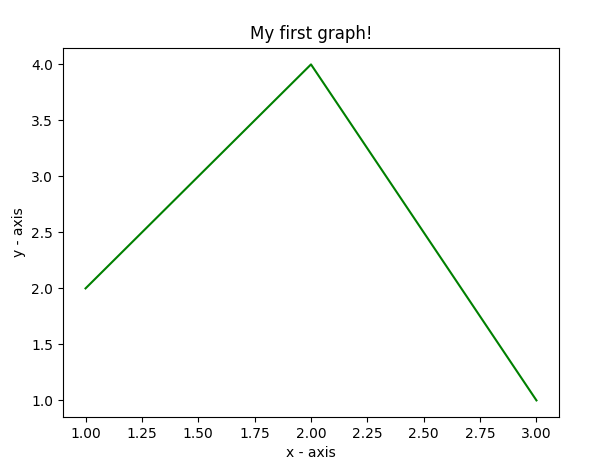The code seems self explanatory. Following steps were followed:

• Define the x-axis and corresponding y-axis values as lists.
• Plot them on canvas using .plot() function.
• Give a name to x-axis and y-axis using .xlabel() and .ylabel() functions.
• Give a title to your plot using .title() function.
• Finally, to view your plot, we use .show() function.

Plotting two or more lines on same plot

 `import` `matplotlib.pyplot as plt`` ` `# line 1 points``x1 ``=` `[``1``,``2``,``3``]``y1 ``=` `[``2``,``4``,``1``]``# plotting the line 1 points ``plt.plot(x1, y1, label ``=` `"line 1"``)`` ` `# line 2 points``x2 ``=` `[``1``,``2``,``3``]``y2 ``=` `[``4``,``1``,``3``]``# plotting the line 2 points ``plt.plot(x2, y2, label ``=` `"line 2"``)`` ` `# naming the x axis``plt.xlabel(``'x - axis'``)``# naming the y axis``plt.ylabel(``'y - axis'``)``# giving a title to my graph``plt.title(``'Two lines on same graph!'``)`` ` `# show a legend on the plot``plt.legend()`` ` `# function to show the plot``plt.show()`

Output: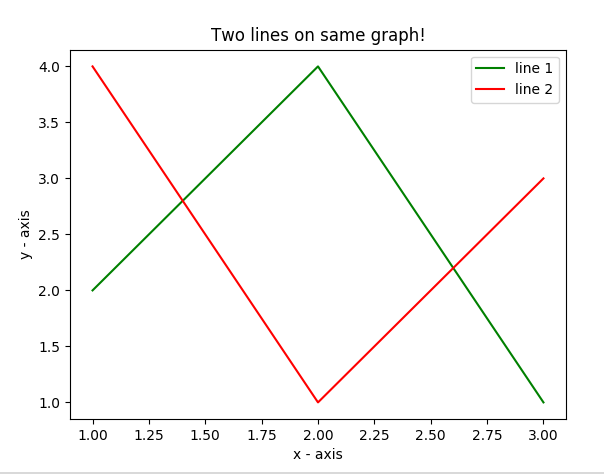• Here, we plot two lines on same graph. We differentiate between them by giving them a name(label) which is passed as an argument of .plot() function.
• The small rectangular box giving information about type of line and its color is called legend. We can add a legend to our plot using .legend() function.

Customization of Plots

Here, we discuss some elementary customizations applicable on almost any plot.

 `import` `matplotlib.pyplot as plt`` ` `# x axis values``x ``=` `[``1``,``2``,``3``,``4``,``5``,``6``]``# corresponding y axis values``y ``=` `[``2``,``4``,``1``,``5``,``2``,``6``]`` ` `# plotting the points ``plt.plot(x, y, color``=``'green'``, linestyle``=``'dashed'``, linewidth ``=` `3``,``         ``marker``=``'o'``, markerfacecolor``=``'blue'``, markersize``=``12``)`` ` `# setting x and y axis range``plt.ylim(``1``,``8``)``plt.xlim(``1``,``8``)`` ` `# naming the x axis``plt.xlabel(``'x - axis'``)``# naming the y axis``plt.ylabel(``'y - axis'``)`` ` `# giving a title to my graph``plt.title(``'Some cool customizations!'``)`` ` `# function to show the plot``plt.show()`

Output: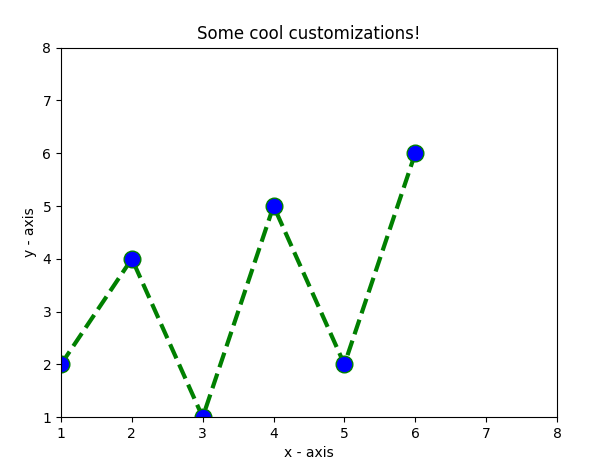As you can see, we have done several customizations like

• setting the line-width, line-style, line-color.
• setting the marker, marker’s face color, marker’s size.
• overriding the x and y axis range. If overriding is not done, pyplot module uses auto-scale feature to set the axis range and scale.

Bar Chart

 `import` `matplotlib.pyplot as plt`` ` `# x-coordinates of left sides of bars ``left ``=` `[``1``, ``2``, ``3``, ``4``, ``5``]`` ` `# heights of bars``height ``=` `[``10``, ``24``, ``36``, ``40``, ``5``]`` ` `# labels for bars``tick_label ``=` `[``'one'``, ``'two'``, ``'three'``, ``'four'``, ``'five'``]`` ` `# plotting a bar chart``plt.bar(left, height, tick_label ``=` `tick_label,``        ``width ``=` `0.8``, color ``=` `[``'red'``, ``'green'``])`` ` `# naming the x-axis``plt.xlabel(``'x - axis'``)``# naming the y-axis``plt.ylabel(``'y - axis'``)``# plot title``plt.title(``'My bar chart!'``)`` ` `# function to show the plot``plt.show()`

Output :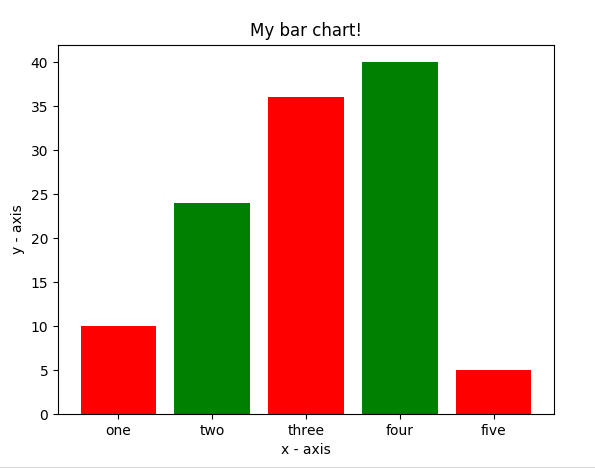• Here, we use plt.bar() function to plot a bar chart.
• x-coordinates of left side of bars are passed along with heights of bars.
• you can also give some name to x-axis coordinates by defining tick_labels

Histogram

 `import` `matplotlib.pyplot as plt`` ` `# frequencies``ages ``=` `[``2``,``5``,``70``,``40``,``30``,``45``,``50``,``45``,``43``,``40``,``44``,``        ``60``,``7``,``13``,``57``,``18``,``90``,``77``,``32``,``21``,``20``,``40``]`` ` `# setting the ranges and no. of intervals``range` `=` `(``0``, ``100``)``bins ``=` `10`  ` ` `# plotting a histogram``plt.hist(ages, bins, ``range``, color ``=` `'green'``,``        ``histtype ``=` `'bar'``, rwidth ``=` `0.8``)`` ` `# x-axis label``plt.xlabel(``'age'``)``# frequency label``plt.ylabel(``'No. of people'``)``# plot title``plt.title(``'My histogram'``)`` ` `# function to show the plot``plt.show()`

Output: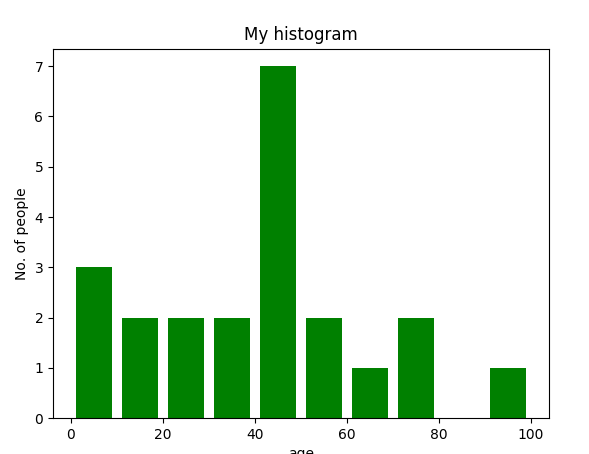• Here, we use plt.hist() function to plot a histogram.
• frequencies are passed as the ages list.
• Range could be set by defining a tuple containing min and max value.
• Next step is to “bin” the range of values—that is, divide the entire range of values into a series of intervals—and then count how many values fall into each interval. Here we have defined bins = 10. So, there are a total of 100/10 = 10 intervals.

Scatter plot

 `import` `matplotlib.pyplot as plt`` ` `# x-axis values``x ``=` `[``1``,``2``,``3``,``4``,``5``,``6``,``7``,``8``,``9``,``10``]``# y-axis values``y ``=` `[``2``,``4``,``5``,``7``,``6``,``8``,``9``,``11``,``12``,``12``]`` ` `# plotting points as a scatter plot``plt.scatter(x, y, label``=` `"stars"``, color``=` `"green"``, ``            ``marker``=` `"*"``, s``=``30``)`` ` `# x-axis label``plt.xlabel(``'x - axis'``)``# frequency label``plt.ylabel(``'y - axis'``)``# plot title``plt.title(``'My scatter plot!'``)``# showing legend``plt.legend()`` ` `# function to show the plot``plt.show()`

Output: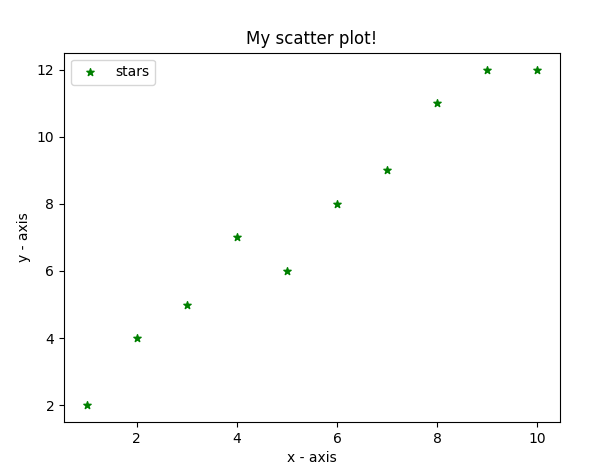• Here, we use plt.scatter() function to plot a scatter plot.
• Like a line, we define x and corresponding y – axis values here as well.
• marker argument is used to set the character to use as marker. Its size can be defined using s parameter.

Pie-chart

 `import` `matplotlib.pyplot as plt`` ` `# defining labels``activities ``=` `[``'eat'``, ``'sleep'``, ``'work'``, ``'play'``]`` ` `# portion covered by each label``slices ``=` `[``3``, ``7``, ``8``, ``6``]`` ` `# color for each label``colors ``=` `[``'r'``, ``'y'``, ``'g'``, ``'b'``]`` ` `# plotting the pie chart``plt.pie(slices, labels ``=` `activities, colors``=``colors, ``        ``startangle``=``90``, shadow ``=` `True``, explode ``=` `(``0``, ``0``, ``0.1``, ``0``),``        ``radius ``=` `1.2``, autopct ``=` `'%1.1f%%'``)`` ` `# plotting legend``plt.legend()`` ` `# showing the plot``plt.show()`

Output of above program looks like this: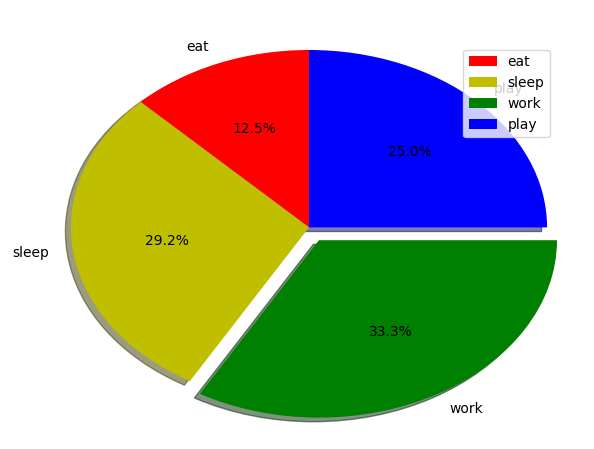• Here, we plot a pie chart by using plt.pie() method.
• First of all, we define the labels using a list called activities.
• Then, portion of each label can be defined using another list called slices.
• Color for each label is defined using a list called colors.
• shadow = True will show a shadow beneath each label in pie-chart.
• startangle rotates the start of the pie chart by given degrees counterclockwise from the x-axis.
• explode is used to set the fraction of radius with which we offset each wedge.
• autopct is used to format the value of each label. Here, we have set it to show the percentage value only upto 1 decimal place.

Plotting curves of given equation

 `# importing the required modules``import` `matplotlib.pyplot as plt``import` `numpy as np`` ` `# setting the x - coordinates``x ``=` `np.arange(``0``, ``2``*``(np.pi), ``0.1``)``# setting the corresponding y - coordinates``y ``=` `np.sin(x)`` ` `# potting the points``plt.plot(x, y)`` ` `# function to show the plot``plt.show()`

Output of above program looks like this: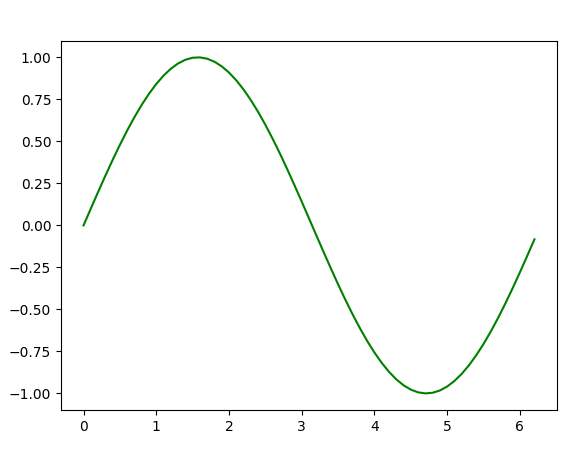Here, we use NumPy which is a general-purpose array-processing package in python.

• To set the x – axis values, we use np.arange() method in which first two arguments are for range and third one for step-wise increment. The result is a numpy array.
• To get corresponding y-axis values, we simply use predefined np.sin() method on the numpy array.
• Finally, we plot the points by passing x and y arrays to the plt.plot() function.

So, in this part, we discussed various types of plots we can create in matplotlib. There are more plots which haven’t been covered but the most significant ones are discussed here –

This article is contributed by Nikhil Kumar. If you like GeeksforGeeks and would like to contribute, you can also write an article using contribute.geeksforgeeks.org or mail your article to contribute@geeksforgeeks.org. See your article appearing on the GeeksforGeeks main page and help other Geeks.

Please write comments if you find anything incorrect, or you want to share more information about the topic discussed above.

Attention geek! Strengthen your foundations with the Python Programming Foundation Course and learn the basics.

To begin with, your interview preparations Enhance your Data Structures concepts with the Python DS Course. And to begin with your Machine Learning Journey, join the Machine Learning – Basic Level Course

My Personal Notes arrow_drop_up